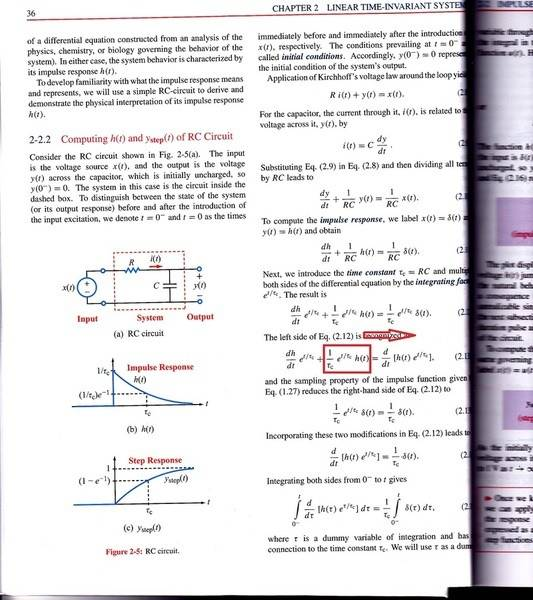# Where did this term go? - Help with Example Problem

Fjolvar
I'm having trouble figuring out how the term below boxed in red was eliminated on the left side of the equation as shown below. I reviewed properties of exponential functions, and still can't understand how this term was cancelled.

1. Homework StatementExponential laws

## The Attempt at a Solution

Well e^(a/b) = b root of e^a which is not the case here. I'm not sure how else this term can be written so that it cancels with the tau c below it in the denominator.

$$\frac{d}{dt} \left[ f(t)g(t) \right] = \frac{df}{dt}g(t)+\frac{dg}{dt}f(t)$$
$$\frac{d}{dt} \left[ h(t) e^{t/{\tau_c}} \right] = \frac{dh}{dt} e^{t/{\tau_c}} + h(t) \frac{d}{dt} \left[ e^{t/{\tau_c}} \right]$$